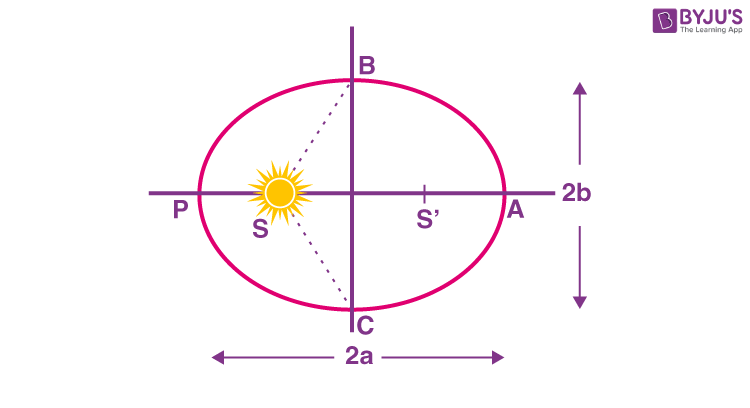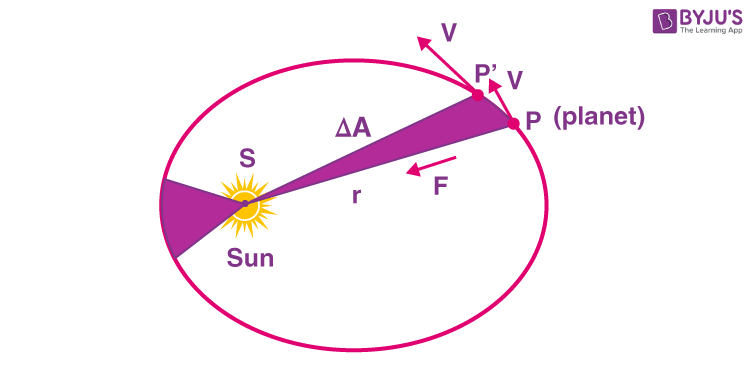Win up to 100% scholarship on Aakash BYJU'S JEE/NEET courses with ABNAT Win up to 100% scholarship on Aakash BYJU'S JEE/NEET courses with ABNAT

# Kepler’s Laws of Planetary Motion

In astronomy, Kepler’s laws of planetary motion are three scientific laws describing the motion of planets around the sun.

• Kepler’s first law – The law of orbits
• Kepler’s second law – The law of equal areas
• Kepler’s third law – The law of periods

## Introduction to Kepler’s Laws

Motion is always relative. Based on the energy of the particle under motion, motions are classified into two types:

• Bounded Motion
• Unbounded Motion

In bounded motion, the particle has negative total energy (E < 0) and has two or more extreme points where the total energy is always equal to the potential energy of the particle, i.e., the kinetic energy of the particle becomes zero.

For eccentricity 0 ≤ e <1, E < 0 implies the body has bounded motion. A circular orbit has eccentricity e = 0, and an elliptical orbit has eccentricity e < 1.

In unbounded motion, the particle has positive total energy (E > 0) and has a single extreme point where the total energy is always equal to the potential energy of the particle, i.e., the kinetic energy of the particle becomes zero.

For eccentricity e ≥ 1, E > 0 implies the body has unbounded motion. The parabolic orbit has eccentricity e = 1, and the hyperbolic path has eccentricity e > 1.

Kepler’s laws of planetary motion can be stated as follows:

## Kepler’s First Law – The Law of Orbits

According to Kepler’s first law, “All the planets revolve around the sun in elliptical orbits having the sun at one of the foci”. The point at which the planet is close to the sun is known as perihelion (about 147 million kilometres from the sun), and the point at which the planet is farther from the sun is known as aphelion (152 million kilometres from the sun). It is characteristic of an ellipse that the sum of the distances of any planet from two foci is constant.Kepler’s First Law – The Law of Orbits

## Kepler’s Second Law – The Law of Equal Areas

Kepler’s second law states, “The radius vector drawn from the sun to the planet sweeps out equal areas in equal intervals of time”.

As the orbit is not circular, the planet’s kinetic energy is not constant in its path. It has more kinetic energy near the perihelion, and less kinetic energy near the aphelion implies more speed at the perihelion and less speed (vmin) at the aphelion. If r is the distance of the planet from the sun at perihelion (rmin) and at aphelion (rmax), then,

rmin + rmax = 2a × (length of the major axis of an ellipse) . . . . . . . (1)Kepler’s Second Law – The Law of Equal Areas

Using the law of conservation of angular momentum, the law can be verified.  At any point of time, the angular momentum can be given as L = mr2ω.

Now, consider a small area ΔA described in a short time interval Δt and the covered angle is Δθ. Let the radius of curvature of the path be r, then the length of the arc covered = r Δθ

ΔA = 1/2[r.(r.Δθ)]= 1/2r2Δθ

Therefore, ΔA/Δt = [ 1/2r2]Δθ/dt

Taking limits on both sides as, Δt→0, we get,

$$\begin{array}{l}\lim_{\Delta t\rightarrow 0}\frac{\Delta A}{\Delta t}=\lim_{\Delta t\rightarrow 0}\frac{1}{2}r^{2}\frac{\Delta \theta }{\Delta t}\end{array}$$
$$\begin{array}{l}\frac{dA}{dt}=\frac{1}{2}r^{2}\omega\end{array}$$
$$\begin{array}{l}\frac{dA}{dt}=\frac{L}{2m}\end{array}$$

Now, by conservation of angular momentum, L is a constant.

Thus, dA/dt = Constant

The area swept in equal intervals of time is a constant.

Kepler’s second law can also be stated as, “The areal velocity of a planet revolving around the sun in elliptical orbit remains constant, which implies the angular momentum of a planet remains constant”. As the angular momentum is constant, all planetary motions are planar motions, which is a direct consequence of central force.

## Kepler’s Third Law – The Law of Periods

According to Kepler’s law of periods,”The square of the time period of revolution of a planet around the sun in an elliptical orbit is directly proportional to the cube of its semi-major axis”.

T2 ∝ a3

The shorter the orbit of the planet around the sun, the shorter the time taken to complete one revolution. Using the equations of Newton’s law of gravitation and laws of motion, Kepler’s third law takes a more general form.

P= 4π2 /[G(M1+ M2)] × a3

Where M1 and M2 are the masses of the two orbiting objects in solar masses.

## Recommended Videos

### Gravitation – Gravitational Potential Energy### Gravitation – Kepler’s Laws of Planetary Motion## Frequently Asked Questions on Kepler’s Law

Q1

### What does Kepler’s first law state?

According to Kepler’s first law, all the planets revolve around the Sun in elliptical orbits, with the Sun as one of the foci.

Q2

### What does Kepler’s second law state?

According to Kepler’s second law, the speed at which the planets move in space continuously changes. The second law helps to explain that when the planets are closer to the Sun, they will travel faster.

Q3

### What is Kepler’s third law?

Kepler’s third law, also called the law of periods, states that the square of the orbital period is proportional to the cube of its mean distance, R.

Q4

### Why are the orbits of the planets not circular?

For the orbits to be circular, it requires the planets to travel with a certain velocity, which is extremely unlikely. If there is any change in the velocity of the planet, the orbit will be elliptical.

Test your Knowledge on Keplers laws